# Curve Fitting and Error Propagation

Absolute Zero: Determination (Example)

Air: Pressure x Temperature (Constant Volume)

Table

t(°C)                 P(cmHg)

0.3200000E+02     0.7330000E+02

0.3500000E+02     0.7450000E+02

0.3800000E+02     0.7510000E+02

0.4100000E+02     0.7600000E+02

0.4400000E+02     0.7680000E+02

0.4700000E+02     0.7760000E+02

0.5000000E+02     0.7820000E+02

0.5300000E+02     0.7900000E+02

0.5600000E+02     0.7960000E+02

0.5900000E+02     0.8020000E+02

0.6200000E+02     0.8100000E+02

0.6500000E+02     0.8150000E+02

0.6800000E+02     0.8210000E+02

0.7100000E+02     0.8270000E+02

Data Information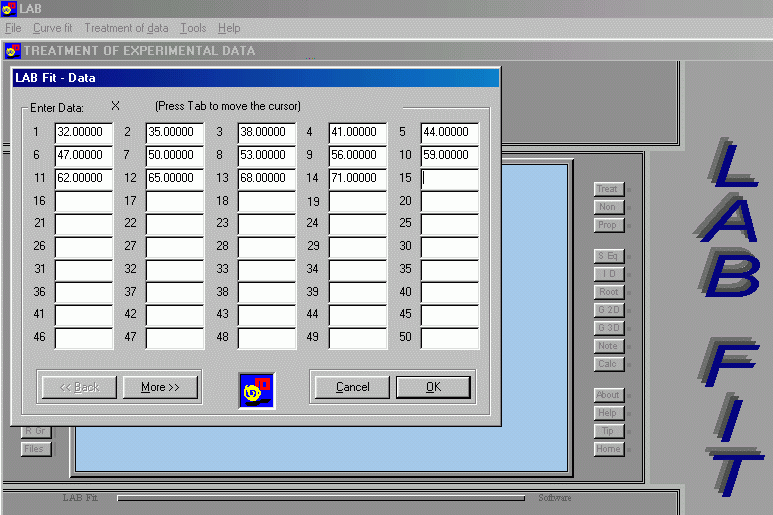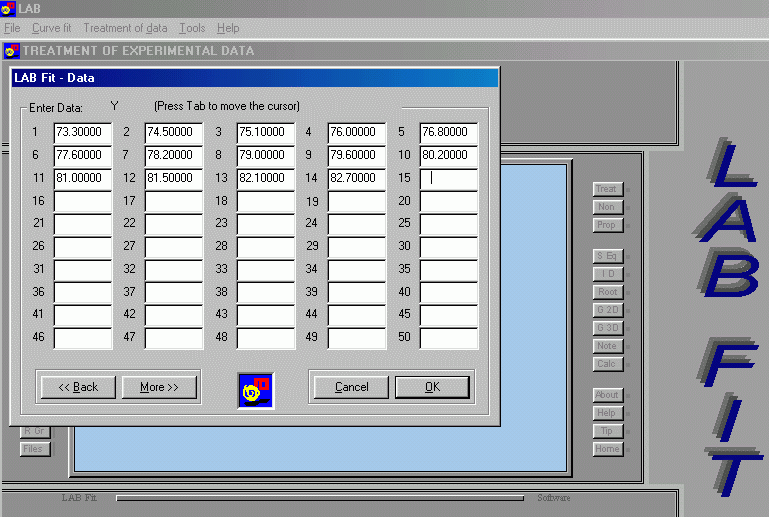Model: P = at + b (y = ax+b)

# See the complete list of library functions (miscellaneous)

Curve Fitting – Detailed Results

(parameters and covariance matrix)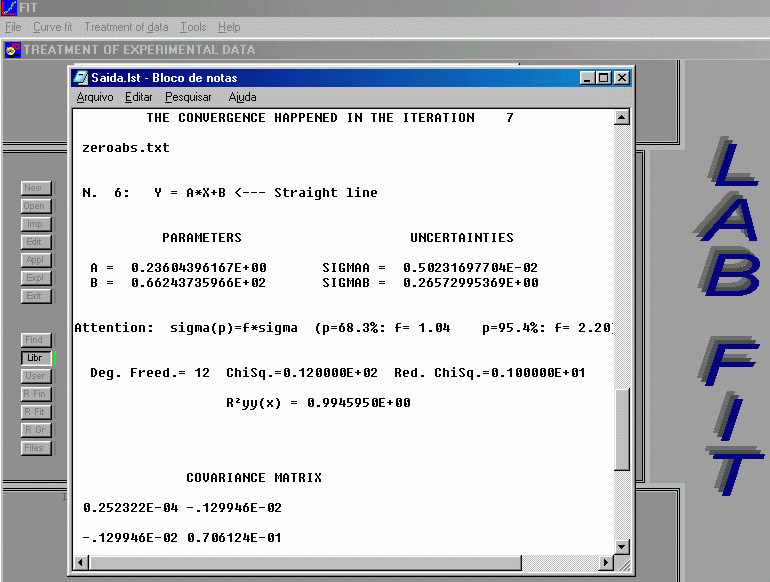Model: P = at + b (y = ax+b)

a = 0.236 ± 0.005;   b = 66.24 ± 0.27   and   cov(a,b) = - .129946E-02

Absolute Zero: Determination  (P = 0)

# P = 0  =>  t = -b/a

## Error Propagation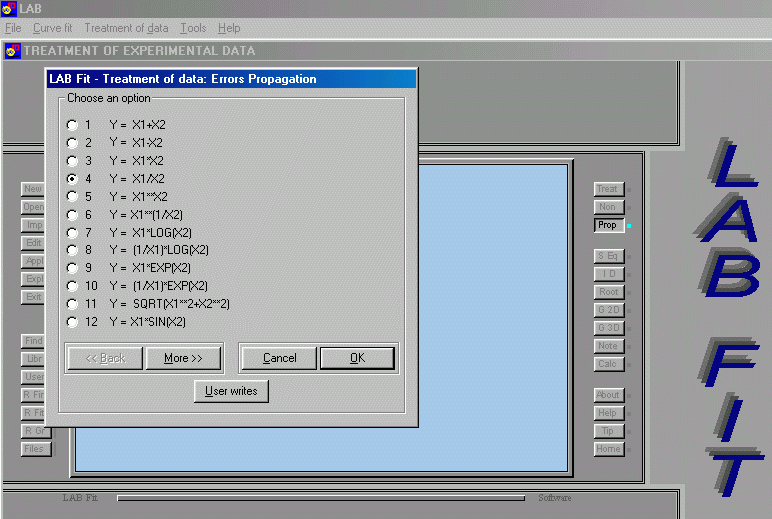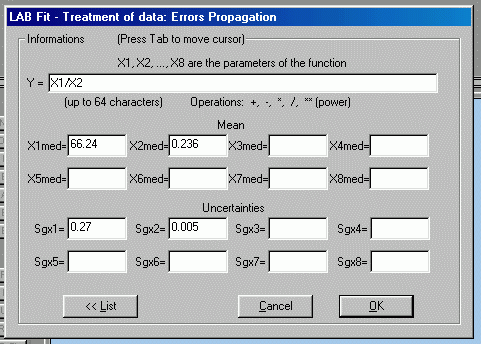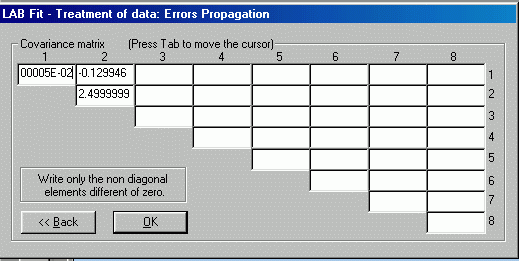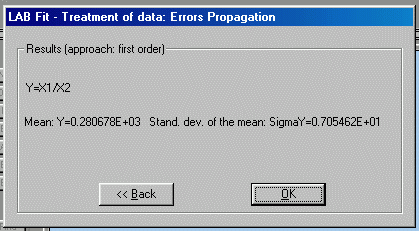| Back |